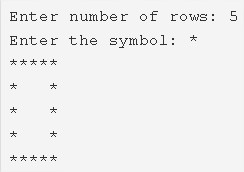# Hollow Square Pattern Program in C | C Programs

C program to print a hollow square pattern – In this article, we will explain the multiple means to print a hollow square pattern in C programming.

Suitable examples and sample programs have also been added so that you can understand the whole thing very clearly. The compiler has also been added with which you can execute it yourself.

The means used in this piece are as follows:

• Using For Loop
• Using While Loop
• Using Do-While Loop

As we all know, Square is a two-dimensional quadrilateral figure which is extremely popular in normal life and geometry as well. A square has equal sides and equal angles, all equal to 90 degrees.

The opposite sides of a square are parallel in nature.As we can see in the image uploaded above, firstly, we need to specify the number of rows the square has to be.

In this case, the number of rows is particularised to be 5.

Later, you have to choose the symbol with which you want to print your square with.

As per the title itself, the ‘*’ symbol is chosen.

Then, the square will be printed as per the code.

Thus, the multiple ways to print a Hollow Square Pattern in C programming are as follows:

## Using For Loop

1. Read the row number and symbol using scanf, getchar() functions and store the values into the variables n, ch.

2) To iterate through rows from i=1 to n run the outer for loop with the structure for(i=1;i<=n;i++).

3) The 2nd for loop iterates through columns from j=1 to n and prints symbols at 1st row, nth row.

4) For remaining rows,the 3rd for loop iterates through columns from j=1 to n with the structure for(j=1;j<=n;j++)

a) prints symbol if j=1 or j=n. Otherwise

b) it prints space.

Output:

### Using While Loop

1. The outer while(i<=n) loop iterates through rows until the condition becomes false.

2) For 1st row, last row

The inner while(j<=n)loop iterates through columns until the condition becomes false.

a) It prints symbol.

b) j value increased by 1(go to while loop).

3) For remaining rows other than the 1st row, last row.

The while(j<=n) loop iterates through columns until the condition becomes false.

a) it prints symbols if j=1 or n.

b) Otherwise, it prints space.

c) j value increased by 1(go to while loop).

2) Cursor comes to next line and i value increased by 1(go to outer while loop).

Output:

#### Using Do-While Loop

1. For i=1 the outer do-while loop iterates the loop one time through rows.

2) If i=1 or i=n, that means at the 1st row or last row.

a) Inner do-while loop iterates through columns and prints the symbol.

b) j value increased by 1.

c) Checks the condition ++j<=n.If condition true, again loop iterates once.Repeats until the condition becomes false.

3) if i!=1 or i!=n, that means other than the 1st row, last row.

a) The inner do-while loop iterates through columns and prints symbol if j=1 or n.

b) Otherwise, it prints space.

c) j value increased by 1.

d) Then checks the condition ++j<=n. If condition true, again the loop iterates once. Repeats until the condition becomes false.

4) a) i value increased by1.

b) Checks the condition i<=n. If the condition is true again the outer loop iterates once. Repeats until the condition becomes false.

Output:

x

## C Program To Print Number Of Days In A Month | Java Tutoring

C program to input the month number and print the number of days in that ...